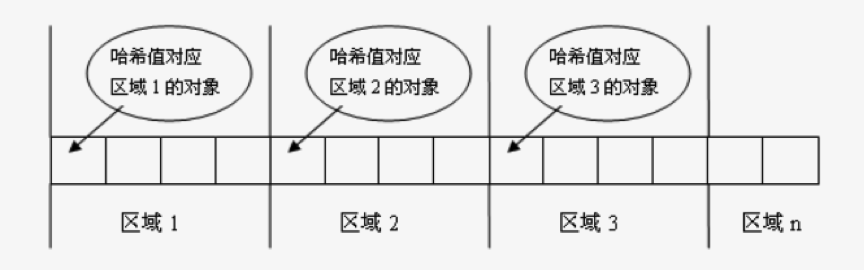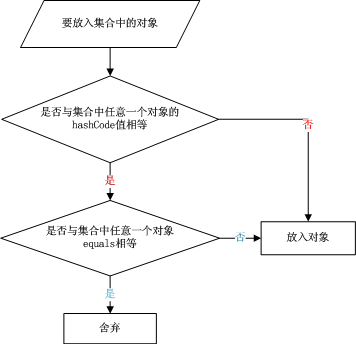# 从一道面试题彻底搞懂hashCode与equals的作用与区别及应当注意的细节

最近去面试了几家公司，被问到hashCode的作用，虽然回答出来了，但是自己还是对hashCode和equals的作用一知半解的，所以决定把它们研究一下。

先来试想一个场景，如果你想查找一个集合中是否包含某个对象，那么程序应该怎么写呢？通常的做法是逐一取出每个元素与要查找的对象一一比较，当发现两者进行equals比较结果相等时，则停止查找并返回true，否则，返回false。但是这个做法的一个缺点是当集合中的元素很多时，譬如有一万个元素，那么逐一的比较效率势必下降很快。于是有人发明了一种哈希算法来提高从该集合中查找元素的效率，这种方式将集合分成若干个存储区域（可以看成一个个桶），每个对象可以计算出一个哈希码，可以根据哈希码分组，每组分别对应某个存储区域，这样一个对象根据它的哈希码就可以分到不同的存储区域（不同的桶中）。如下图所示：hashCode 的常规协定是：

1.若重写了equals(Object obj)方法，则有必要重写hashCode()方法。

2.若两个对象equals(Object obj)返回true，则hashCode（）有必要也返回相同的int数。

3.若两个对象equals(Object obj)返回false，则hashCode（）不一定返回不同的int数。

4.若两个对象hashCode（）返回相同int数，则equals（Object obj）不一定返回true。

5.若两个对象hashCode（）返回不同int数，则equals（Object obj）一定返回false。

6.同一对象在执行期间若已经存储在集合中，则不能修改影响hashCode值的相关信息，否则会导致内存泄露问题。HashSet.java

  public boolean add(E e) {
return map.put(e, PRESENT)==null;
}

HashMap.java

    public V put(K key, V value) {
if (key == null)
return putForNullKey(value);
int hash = hash(key.hashCode());
int i = indexFor(hash, table.length);
for (Entry<K,V> e = table[i]; e != null; e = e.next) {
Object k;
if (e.hash == hash && ((k = e.key) == key || key.equals(k))) {
V oldValue = e.value;
e.value = value;
e.recordAccess(this);
return oldValue;
}
}

modCount++;
return null;
}

public class HashCodeTest {
public static void main(String[] args) {
Collection set = new HashSet();
Point p1 = new Point(1, 1);
Point p2 = new Point(1, 1);

System.out.println(p1.equals(p2));

Iterator iterator = set.iterator();
while (iterator.hasNext()) {
Object object = iterator.next();
System.out.println(object);
}
}
}

class Point {
private int x;
private int y;

public Point(int x, int y) {
super();
this.x = x;
this.y = y;
}

@Override
public boolean equals(Object obj) {
if (this == obj)
return true;
if (obj == null)
return false;
if (getClass() != obj.getClass())
return false;
Point other = (Point) obj;
if (x != other.x)
return false;
if (y != other.y)
return false;
return true;
}

@Override
public String toString() {
return "x:" + x + ",y:" + y;
}

}


true
x:1,y:1
x:1,y:1


class Point {
private int x;
private int y;

public Point(int x, int y) {
super();
this.x = x;
this.y = y;
}

@Override
public int hashCode() {
final int prime = 31;
int result = 1;
result = prime * result + x;
result = prime * result + y;
return result;
}

@Override
public String toString() {
return "x:" + x + ",y:" + y;
}

}


false
x:1,y:1
x:1,y:1

（注意：在HashSet中插入同一个元素（hashCode和equals均相等）时，会被舍弃，而在HashMap中插入同一个Key（Value 不同）时，原来的元素会被覆盖。）

public class HashCodeTest {
public static void main(String[] args) {
Collection set = new HashSet();
Point p1 = new Point(1, 1);
Point p2 = new Point(1, 2);

p2.setX(10);
p2.setY(10);

set.remove(p2);

Iterator iterator = set.iterator();
while (iterator.hasNext()) {
Object object = iterator.next();
System.out.println(object);
}
}
}

class Point {
private int x;
private int y;

public Point(int x, int y) {
super();
this.x = x;
this.y = y;
}

public int getX() {
return x;
}

public void setX(int x) {
this.x = x;
}

public int getY() {
return y;
}

public void setY(int y) {
this.y = y;
}

@Override
public int hashCode() {
final int prime = 31;
int result = 1;
result = prime * result + x;
result = prime * result + y;
return result;
}

@Override
public boolean equals(Object obj) {
if (this == obj)
return true;
if (obj == null)
return false;
if (getClass() != obj.getClass())
return false;
Point other = (Point) obj;
if (x != other.x)
return false;
if (y != other.y)
return false;
return true;
}

@Override
public String toString() {
return "x:" + x + ",y:" + y;
}

}


x:1,y:1
x:10,y:10

假设p1的hashCode为1，p2的hashCode为2，在存储时p1被分配在1号桶中，p2被分配在2号筒中。这时修改了p2中与计算hashCode有关的信息（x和y）,当调用remove(Object obj)时，首先会查找该hashCode值得对象是否在集合中。假设修改后的hashCode值为10（仍存在2号桶中）,这时查找结果空，jdk认为该对象不在集合中，所以不会进行删除操作。然而用户以为该对象已经被删除，导致该对象长时间不能被释放，造成内存泄露。解决该问题的办法是不要在执行期间修改与hashCode值有关的对象信息，如果非要修改，则必须先从集合中删除，更新信息后再加入集合中。

1.hashCode是为了提高在散列结构存储中查找的效率，在线性表中没有作用。
2.equals和hashCode需要同时覆盖。
3.若两个对象equals返回true，则hashCode有必要也返回相同的int数。

4.若两个对象equals返回false，则hashCode不一定返回不同的int数,但为不相等的对象生成不同hashCode值可以提高 哈希表的性能。

5.若两个对象hashCode返回相同int数，则equals不一定返回true。

6.若两个对象hashCode返回不同int数，则equals一定返回false。

7.同一对象在执行期间若已经存储在集合中，则不能修改影响hashCode值的相关信息，否则会导致内存泄露问题。

06-081万+03-038666
09-205万+
07-125万+
06-026万+
07-315147
05-15445
08-122万+